# 阻塞队列源码系列（四）：PriorityBlockingQueue

Posted by 陈树义 on 2021-06-20

PriorityBlockingQueue，是在JDK1.5时，随着J.U.C包引入的一种阻塞队列，它实现了 BlockingQueue 接口，底层基于堆实现：## 特性

PriorityBlockingQueue 是一种无界阻塞队列，在构造的时候可以指定队列的初始容量，也可以不指定其容量。当队列容量不够时，其会自动扩容，除非达到最大值，否则会一直扩容下去。

• PriorityBlockingQueue 与之前介绍的阻塞队列最大的不同之处就是：它是一种优先级队列，也就是说元素并不是以 FIFO 的方式出/入队，而是以按照权重大小的顺序出队。
• PriorityBlockingQueue 表面上看是无界队列，但其有最大容量的限制（Integer.MAX_VALUE - 8）。只是其在初始化时并不会限制其最大容量，而是在需要的时候自动扩容。
• 由于 PriorityBlockingQueue 是按照元素的权重进入排序，所以队列中的元素必须是可以比较的，也就是说元素必须实现 Comparable 接口。
• 由于 PriorityBlockingQueue 无界队列，所以插入元素永远不会阻塞线程。

PriorityBlockingQueue底层是一种基于数组实现的堆结构。

## 原理

### 类成员变量

``````// 默认容量大小
private static final int DEFAULT_INITIAL_CAPACITY = 11;
// 最大容量大小
private static final int MAX_ARRAY_SIZE = Integer.MAX_VALUE - 8;
// 保存数据的数组
private transient Object[] queue;
// 队列容量
private transient int size;
// 比较器
private transient Comparator<? super E> comparator;
// 并发锁
private final ReentrantLock lock;
private final Condition notEmpty;
``````

• PriorityBlockingQueue 底层使用数组实现（其实是一个二叉堆）。
• PriorityBlockingQueue 使用单锁单条件（lock）实现并发控制，因为会自动扩容，所以其只有一个 notEmpty 条件。

### 构造方法

``````public PriorityBlockingQueue() {
this(DEFAULT_INITIAL_CAPACITY, null);
}
``````
``````public PriorityBlockingQueue(int initialCapacity) {
this(initialCapacity, null);
}
``````
``````public PriorityBlockingQueue(int initialCapacity,
Comparator<? super E> comparator) {
if (initialCapacity < 1)
throw new IllegalArgumentException();
this.lock = new ReentrantLock();
this.notEmpty = lock.newCondition();
this.comparator = comparator;
this.queue = new Object[initialCapacity];
}
``````
``````public PriorityBlockingQueue(Collection<? extends E> c) {
this.lock = new ReentrantLock();
this.notEmpty = lock.newCondition();
boolean heapify = true; // true if not known to be in heap order
boolean screen = true;  // true if must screen for nulls
// 1.判断是会否是特殊集合
if (c instanceof SortedSet<?>) {
SortedSet<? extends E> ss = (SortedSet<? extends E>) c;
this.comparator = (Comparator<? super E>) ss.comparator();
heapify = false;
}
else if (c instanceof PriorityBlockingQueue<?>) {
PriorityBlockingQueue<? extends E> pq =
(PriorityBlockingQueue<? extends E>) c;
this.comparator = (Comparator<? super E>) pq.comparator();
screen = false;
if (pq.getClass() == PriorityBlockingQueue.class) // exact match
heapify = false;
}
Object[] a = c.toArray();
int n = a.length;
// If c.toArray incorrectly doesn't return Object[], copy it.
if (a.getClass() != Object[].class)
a = Arrays.copyOf(a, n, Object[].class);
if (screen && (n == 1 || this.comparator != null)) {
for (int i = 0; i < n; ++i)
if (a[i] == null)
throw new NullPointerException();
}
this.queue = a;
this.size = n;
// 2.调用 heapify 方法对元素进行排序
if (heapify)
heapify();
}
``````

• 首先，判断集合是否是特殊的集合（SortedSet、PriorityBlockingQueue）。如果是特殊集合，那么就可以不需要重新排序。
• 最后，调用 heapify 方法对元素进行排序。

``````private void heapify() {
Object[] array = queue;
int n = size;
int half = (n >>> 1) - 1;
Comparator<? super E> cmp = comparator;
if (cmp == null) {
for (int i = half; i >= 0; i--)
siftDownComparable(i, (E) array[i], array, n);
}
else {
for (int i = half; i >= 0; i--)
siftDownUsingComparator(i, (E) array[i], array, n, cmp);
}
}
``````

### 核心方法

• offer/poll
• put/take

``````public boolean add(E e) {
return offer(e);
}
``````

``````public boolean remove(Object o) {
final ReentrantLock lock = this.lock;
lock.lock();
try {
int i = indexOf(o);
if (i == -1)
return false;
removeAt(i);
return true;
} finally {
lock.unlock();
}
}
``````

``````private void removeAt(int i) {
Object[] array = queue;
int n = size - 1;
if (n == i) // removed last element
array[i] = null;
else {
E moved = (E) array[n];
array[n] = null;
Comparator<? super E> cmp = comparator;
if (cmp == null)
siftDownComparable(i, moved, array, n);
else
siftDownUsingComparator(i, moved, array, n, cmp);
if (array[i] == moved) {
if (cmp == null)
siftUpComparable(i, moved, array);
else
siftUpUsingComparator(i, moved, array, cmp);
}
}
size = n;
}
``````

removeAt 方法的逻辑大致为：首先，判断是否移除最后一个元素。如果移除的是最后一个元素，那么直接将其置为 null 即可。如果移除的并不是最后一个元素，那么就必须对二叉树数组进行维护。我们继续看看 siftDownComparable 方法。

``````private static <T> void siftDownComparable(int k, T x, Object[] array,
int n) {
if (n > 0) {
Comparable<? super T> key = (Comparable<? super T>)x;
int half = n >>> 1;           // loop while a non-leaf
while (k < half) {
int child = (k << 1) + 1; // assume left child is least
Object c = array[child];
int right = child + 1;
if (right < n &&
((Comparable<? super T>) c).compareTo((T) array[right]) > 0)
c = array[child = right];
if (key.compareTo((T) c) <= 0)
break;
array[k] = c;
k = child;
}
array[k] = key;
}
}
``````

• 找到该顶点的左右子结点中较小的那个。
• 与当前结点交换。
• 重复前2步直到当前结点没有左右子结点或比左右子结点都小。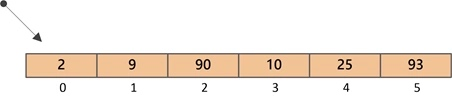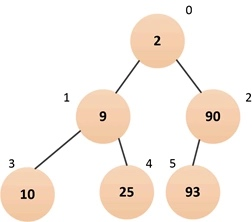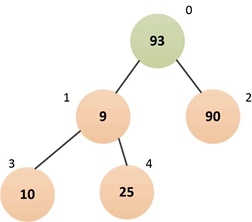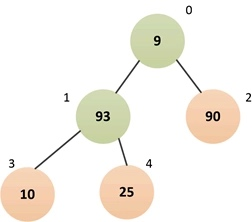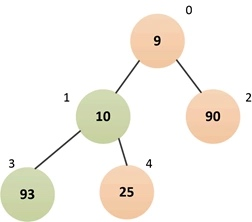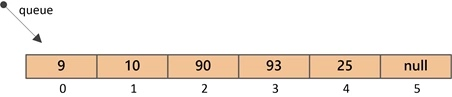#### offer/poll方法

``````public boolean offer(E e) {
if (e == null)
throw new NullPointerException();

final ReentrantLock lock = this.lock;   // 加锁
lock.lock();

int n, cap;
Object[] array;
while ((n = size) >= (cap = (array = queue).length))    // 队列已满, 则进行扩容
tryGrow(array, cap);

try {
Comparator<? super E> cmp = comparator;
if (cmp == null)    // 比较器为空, 则按照元素的自然顺序进行堆调整
siftUpComparable(n, e, array);
else                // 比较器非空, 则按照比较器进行堆调整
siftUpUsingComparator(n, e, array, cmp);
size = n + 1;       // 队列元素总数+1
notEmpty.signal();  // 唤醒一个可能正在等待的"出队线程"
} finally {
lock.unlock();
}
return true;
}
``````

``````/**
* 将元素x插入到array[k]的位置.
* 然后按照元素的自然顺序进行堆调整——"上浮"，以维持"堆"有序.
* 最终的结果是一个"小顶堆".
*/
private static <T> void siftUpComparable(int k, T x, Object[] array) {
Comparable<? super T> key = (Comparable<? super T>) x;
while (k > 0) {
int parent = (k - 1) >>> 1;     // 相当于(k-1)除2, 就是求k结点的父结点索引parent
Object e = array[parent];
if (key.compareTo((T) e) >= 0)  // 如果插入的结点值大于父结点, 则退出
break;

// 否则，交换父结点和当前结点的值
array[k] = e;
k = parent;
}
array[k] = key;
}
``````

siftUpComparable方法的作用其实就是堆的“上浮调整”，可以把堆可以想象成一棵完全二叉树，每次插入元素都链接到二叉树的最右下方，然后将插入的元素与其父结点比较，如果父结点大，则交换元素，直到没有父结点比插入的结点大为止。这样就保证了堆顶（二叉树的根结点）一定是最小的元素（注：以上仅针对“小顶堆”）。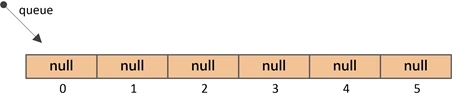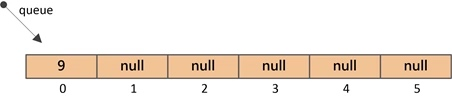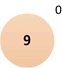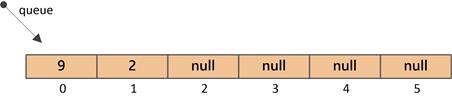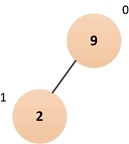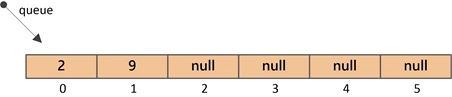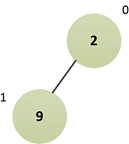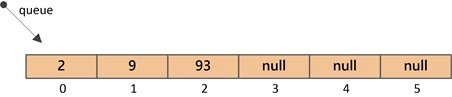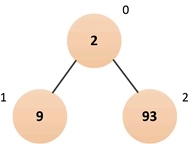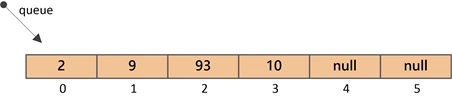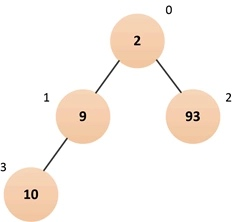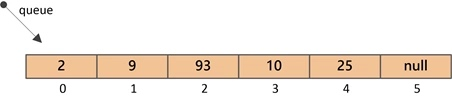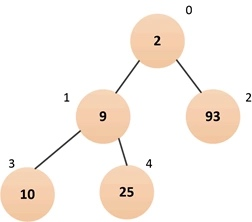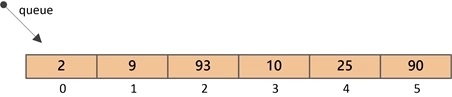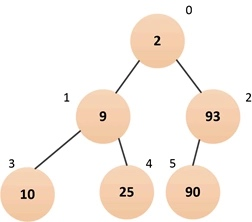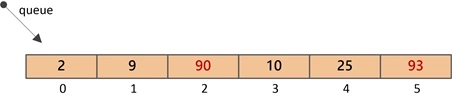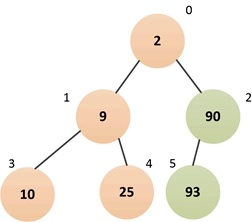``````public E poll() {
final ReentrantLock lock = this.lock;
lock.lock();
try {
return dequeue();
} finally {
lock.unlock();
}
}
``````

``````private E dequeue() {
int n = size - 1;
if (n < 0)
return null;
else {
Object[] array = queue;
E result = (E) array;
E x = (E) array[n];
array[n] = null;
Comparator<? super E> cmp = comparator;
if (cmp == null)
siftDownComparable(0, x, array, n);
else
siftDownUsingComparator(0, x, array, n, cmp);
size = n;
return result;
}
}
``````

#### put/take方法

put 方法的实现，其实是调用了 offer 方法，这里也不再赘述。

``````public void put(E e) {
offer(e); // never need to block
}
``````

take 方法的实现，其实是调用了 dequeue 方法。

``````public E take() throws InterruptedException {
final ReentrantLock lock = this.lock;
lock.lockInterruptibly();
E result;
try {
while ( (result = dequeue()) == null)
notEmpty.await();
} finally {
lock.unlock();
}
return result;
}
``````

#### 扩容

``````public boolean offer(E e) {

// ...

while ((n = size) >= (cap = (array = queue).length))    // 队列已满, 则进行扩容
tryGrow(array, cap);

// ...
}
``````

``````private void tryGrow(Object[] array, int oldCap) {
// 1.释放全局锁
lock.unlock();  // 扩容和入队/出队可以同时进行, 所以先释放全局锁
Object[] newArray = null;
// 2.设置扩容标识字段
if (allocationSpinLock == 0 &&
UNSAFE.compareAndSwapInt(this, allocationSpinLockOffset,
0, 1)) {    // allocationSpinLock置1表示正在扩容
try {
// 3.计算新的数组大小
int newCap = oldCap + ((oldCap < 64) ?
(oldCap + 2) :
(oldCap >> 1));
if (newCap - MAX_ARRAY_SIZE > 0) {    // 溢出判断
int minCap = oldCap + 1;
if (minCap < 0 || minCap > MAX_ARRAY_SIZE)
throw new OutOfMemoryError();
newCap = MAX_ARRAY_SIZE;
}
if (newCap > oldCap && queue == array)
newArray = new Object[newCap];  // 分配新数组
} finally {
allocationSpinLock = 0;
}
}
if (newArray == null)   // 扩容失败（可能有其它线程正在扩容，导致allocationSpinLock竞争失败）
// 4.获取全局锁并开始复制数组
lock.lock();            // 获取全局锁(因为要修改内部数组queue)
if (newArray != null && queue == array) {
queue = newArray;   // 指向新的内部数组
System.arraycopy(array, 0, newArray, 0, oldCap);
}
}
``````

## 总结